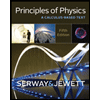# A disk, with a radius of 0.25 m, is to be rotated like a merry-go-round through 800 rad, starting from rest, gaining angular speed at the constant rate α1 through the first 400 rad and then losing angular speed at the constant rate -α1 until it is again at rest. The magnitude of the centripetal acceleration of any portion of the disk is not to exceed 100 m/s2. (a) What is the least time required for the rotation? (b) What is the corresponding value of α1?

Question

A disk, with a radius of 0.25 m, is to be rotated like a merry-go-round through 800 rad, starting from rest, gaining angular speed at the constant rate α1 through the first 400 rad and then losing angular speed at the constant rate -α1 until it is again at rest. The magnitude of the centripetal acceleration of any portion of the disk is not to exceed 100 m/s2. (a) What is the least time required for the rotation? (b) What is the corresponding value of α1?

Expert Solution

### Want to see the full answer?

Check out a sample Q&A hereStudents who’ve seen this question also like:Principles of Physics: A Calculus-Based Text
5th Edition
ISBN: 9781133104261
Author: Raymond A. Serway, John W. Jewett
Publisher: Cengage Learning